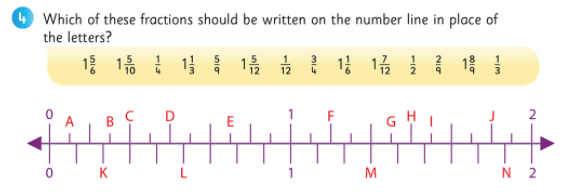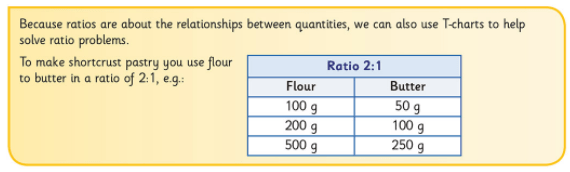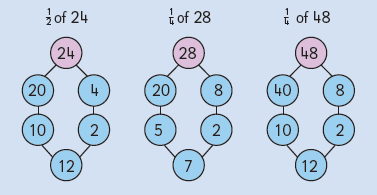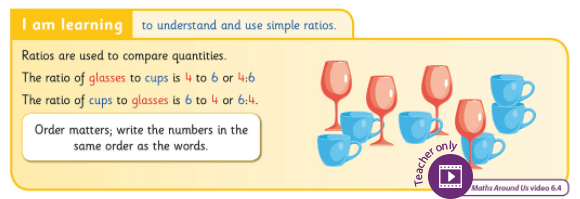# Monthly Archives: December 2017

## Digging Deeper into … Fractions (1st – 6th)

Category : Uncategorized

For practical suggestions for families, and links to useful digital resources, to support children learning about the topic of fractions, please check out the following post: Dear Family, your Operation Maths Guide to Fractions

‘The headlong rush into computation with fractions, using such mumbo-jumbo as “add the tops but not the bottoms” or “turn it upside-down and multiply”, has often been attempted before the idea of a fraction or fractional notation has been fully understood.’

Nuffield Maths 3 Teachers’ Handbook, Longman 1991

Fractions, as is often acknowledged, can be a very problematic topic; so much of this concept appears to be at odds with key concepts in other areas, eg:

• Children’s understanding of number tells them that 4 is greater than 2, yet 1/4 is not greater than 1/2 of the same shape. Similarly, fractions cannot be ordered using whole number understanding.
• The size/value of a fraction depends on the size/value of the whole from which the fraction is taken, i.e. a quarter of one number, e.g. 100, can be greater than a half of another number, e.g. 30. From the beginning, it’s important to stress that fractions are not whole numbers, but rather, they represent bits or parts of a whole and that the whole can be different each time.
•Equivalent fractions of the same shape do not always have to be the same shape (congruent), as can be seen from the quarters of the square in the example opposite, but each quarter should have the same area.
• Fractional language and terminology can pose difficulties. Verbally there is little to distinguish between eighth and eight, ten and tenth, etc. In addition, eighth can be one whole cut into eight equal pieces, and as an ordinal number it represents the position between seventh and ninth.

Thus, not only is it very important that the children appreciate the importance of accuracy when communicating about fractions, it is essential that children are given sufficient opportunities to develop a solid understanding of fractions: what they are, how they are made, changed, used, etc. Therefore, as typical in Operation Maths, the activities in this topic follow a concrete–pictorial–abstract approach.

### Variety of representations

In Operation Maths, a variety of activity types are incorporated, based on linear, area (shapes) and set models. It is very important that children use a variety of materials and representations when exploring fractions, as otherwise they may only be able to relate their fractional understanding to a certain model.

• Area models: includes dividing shapes such as circles, squares, rectangles etc into various fractions and/or using these type of representations.
• Linear models: includes using strips of cubes to show fractions, marking fractions on a number line, comparing fractions on a fraction wall, using bar models
• Set models: Dividing a set of identical objects or a number into halves, quarters etc; identifying or calculating the whole amount when a fraction of the whole is known etc.For more information on the various types of fractions models watch any of the videos at the links below:

In keeping with the overall CPA approach of Operation Maths, the initial part of the fraction chapters will focus on the children having plenty of experiences using concrete materials (e.g. creating fractions using the various models, comparing and ordering them) and/or interacting with pictorial representations (e.g. combining and partitioning fractions and labelling them using the appropriate names and symbols). It is important that the children have sufficient experiences at these levels before progressing to more abstract tasks e.g. calculating with fractions.

In the Operation Maths TRBs there are a multitude of suggested tasks, including fraction stations, based on the exploration and use of concrete manipulatives. Other suitable concrete-based activities include using Lego and/or pattern blocks to explore fractions.

Problem solving strategies that have been used previously to promote visualisation, should also be incorporated into this topic, including bar models, empty number lines and T-charts (see images below).### Calculating fractions

When calculating a fraction of an amount, or calculating the whole amount given a fraction, bar models can be used to represent the information and solve for the answer (see bar models above). The children should also be encouraged to develop and use strategies to calculate mentally where possible, for example partition the amount into friendly numbers (using branching, if written, as shown below) and then re-combine.### Operations with fractions (5th & 6th)

Fifth class is the first time that children will formally encounter operations involving fractions. The curriculum for fifth class requires that the children be enabled to ‘add and subtract simple fractions and simple mixed numbers’ and to ‘multiply a fraction by a whole number’. The emphasis here should be on the word simple, and the simplest fractions for most children are likely to be halves, quarters and eighths, since these are the fractions the children are most likely to encounter in real life, as well as being among the earliest fractions they met in primary mathematics. Therefore, it is sufficient for some children to be enabled to achieve this objective using only wholes, halves, quarters and eighths. Other more-able children should be allowed to extend their understanding to other fractions, e.g. fifths and tenths, then thirds, sixths, ninths and twelfths, and finally to unconnected fractions if relevant, such as sevenths and elevenths.Strategies that are used in Operation Maths for addition and subtraction of whole numbers, (and that are used in Number Talks) can often be applicable for addition and subtraction using fractions also e.g.:

• Compensation: moving an amount from one addend to the other addend to make a more friendly (compatible) number e.g. in example A above, moving 6/10 to 2/5 to make 1 whole unit.
• Partitioning: separating the whole numbers from the fractions and adding each separately, before recombining, as shown in example B above.

Similarly for subtraction, fraction number lines can be used to model either the taking away (deduction) strategy or the difference (adding up) strategy. A selection of number lines for this very purpose are provided on the inside back cover of the Operation Maths pupils books for 5th and 6th classes.

### Ratios

Sixth class is the first time that children will formally encounter ratios. It is important that they realise that ratios don’t necessarily tell you the quantity of items in a group/set, rather how many of one quantity there is to an amount of another quantity. For example the ratio of teachers to pupils in the school is 1:27. That doesn’t tell us that there is only one teacher or just 27 students in the school, rather that there is a teacher for every 27 students.Avoid relying on rules such as ‘divide by the bottom, multiply by the top’, ‘add/subtract the tops but not the bottoms’, ‘multiply tops and bottoms‘. These rules are very abstract and do not encourage the children to visualise the numbers or fractions involved and what is being asked. Such rules also reinforce incorrectly that teaching maths is about teaching the children to do maths, rather than teaching them to understand maths. And, even if such rules are used to calculate correctly, it can often be in the wrong situation, e.g. ‘What is the whole number if 3/4 is 12’, to which the child “divides by the bottom, multiplies by the top” to give an incorrect answer of 9.

Rather, the emphasis should be on the children developing the ability to visualise fractions and fractions of sets/numbers. Using concrete materials and pictorial representations (e.g. fraction pie pieces, number lines, arrays, bar model drawings, etc.) can greatly aid this. Ultimately, it is hoped that the children will identify some shortcuts themselves (e.g. simplifying the multiplication of fractions), that may echo the traditional fraction rules; however, the emphasis needs to be on these arising from the children’s own discoveries.

• Dear Family, your Operation Maths Guide to Fractions includes practical suggestions for supporting children, and links to a huge suite of digital resources, organised according to class level.
• Virtual Maths Manipulatives for Fractions, Decimals and Percentages: Lots of tools that can be used in many different ways to explore these concepts.
• Operation Maths users don’t forget to check out the extensive digital resources available for this topic on Edco Learning. These include Maths Around Us and Write, Hide, Show videos, and create Create Activities using the Fraction eManipulative, sorting eManipulative and bar modelling eManipulative. Operation Maths users in 3rd to 6th should check out the first page of the Fractions chapter in their Pupils Books for a quick synopsis of the suggested digital resources and thne refer to their TRB for more detailed information.
• Check out this Pinterest Board of Fraction ideas
• Fractions Manual from PDST
• Fraction Models: an informative article with images and teaching ideas, from K-5 Math
• NRICH: selection of problems, articles and games for fractions
• Watch any of these informative videos: